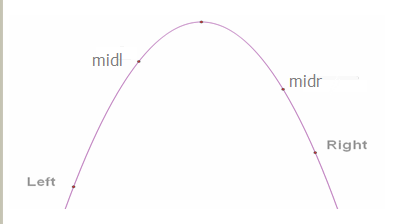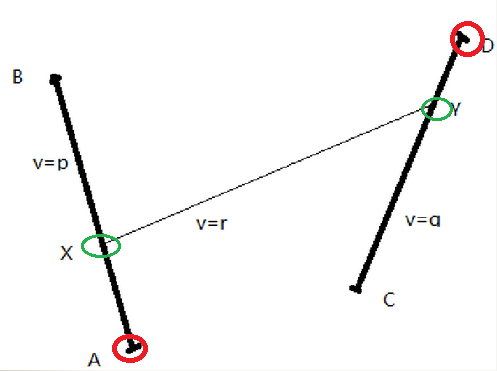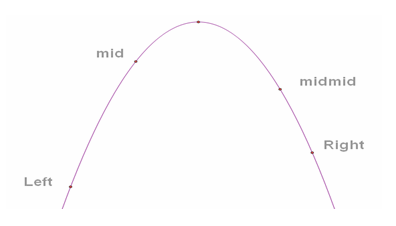• 三分法 当需要求某凸性或凹形函数的极值，通过函数本身表达式并不容易求解时，就可以用三分法不断逼近求解。 类似二分的定义Left和Right mid = (Left + Right) / 2 midmid = (mid + Right) / 2; 如果mid靠近极值点，...
三分法 当需要求某凸性或凹形函数的极值，通过函数本身表达式并不容易求解时，就可以用三分法不断逼近求解。 类似二分的定义Left和Right mid = (Left + Right) / 2 midmid = (mid + Right) / 2; 如果mid靠近极值点，则Right = midmid； 否则(即midmid靠近极值点)，则Left = mid; 当需要作用二分法三分法的时候，对于求解一些实际问题，当公式难以推导出来时，二分、三分法可以较为精确地求解出一些临界值，且效率也是令人满意的。灵活应用这些方法对解题会很有帮助。
展开全文• 这里是ACM学习笔记（1）二分法、三分法。每次学习笔记都将会以思维导图等多种灵活形式展示出来，这里面的博客和习题都是属于精选，并且会不定时更新完善笔记。ACM学习笔记（0）总纲要 总括 二分法（一）：二分法的...
这里是ACM学习笔记（1）二分法、三分法。每次学习笔记都将会以思维导图等多种灵活形式展示出来，这里面的博客和习题都是属于精选，并且会不定时更新完善笔记。ACM学习笔记（0）总纲要
总括
二分法（一）：二分法的基本思想
应用
快速幂
模板 | 整数快速幂 & 快速幂取模
模板题
P1226 【模板】快速幂||取余运算
矩阵快速幂
矩阵快速幂总结 快速幂+矩阵快速幂（总结+例题）
模板题
P3390 【模板】矩阵快速幂 P1962 斐波那契数列 P1939 【模板】矩阵加速（数列）
二分查找
详解二分查找算法 你真的会写二分查找吗 你真的了解二分查找吗？
二分答案
三种常见的二分答案模板 二分法（二）：采用二分法解决“最小化最大值问题” 二分法（三）：采用二分法解决“最大化最小值问题” 二分法（四）：采用二分法解决“最大化平均值”问题 二分答案总结 二分答案模板及二分答案问题讲解 浅谈三分法的原理及应用
展开全文二分法 算法
• ## ACM-三分搜索

千次阅读 2015-03-24 21:21:36
类似于二分查找，三分搜索法也是比较常用的基于分治思想的高效查找方法。...但是为什么三分法可以用于凸函数或者凹函数呐，这其实是因为这种函数总是有一个最大值或者最小值，这样就可以借此判断出三分法中两个中点相
类似于二分查找，三分搜索法也是比较常用的基于分治思想的高效查找方法。但是和二分不同，二分只适用于单调函数，比如常用的对单调递增或单调递减的一个序列中的某一个元素进行查找，三分却突破了这种限制，可以用于左边递增右边递减或者相反的，这么一类函数，也就是常说的凸函数和凹函数。但是为什么三分法可以用于凸函数或者凹函数呐，这其实是因为这种函数总是有一个最大值或者最小值，这样就可以借此判断出三分法中两个中点相对相对于极值的位置，例如下图（凹函数类似）：三分搜索的实现主要是判断midl和midr所在值的大小。以凸函数为例（凹函数类似，只是判mid大小的时候保留小的即可（其实也是保留离极值最近的mid）），先以left和right为端点计算出它们的中点midl，然后再以midl和right为端点计算出它们的中点midr，接下来就需要判断f(midl)和f(midr)值的大小了，如果f(midl)大于f(midr)，那么说明midl靠近极值，此时令right=midr，否则说明midr靠近极值，此时则令left=midl，总之就是要保留离极值最近的那一个mid，然后重复前面的过程，直到left和right十分接近，最终f(left)就等于了极值，下面给出程序实现：

double solve(double parameter)
{
// 计算函数值，即f(x)
}

double trisection_search(double left, double right)
{
// 三分搜索，找到最优解（求函数最大值下的自变量值）
double midl, midr;
while (right-left > 1e-7)
{
midl = (left + right) / 2;
midr = (midl + right) / 2;
// 如果是求最小值的话这里判<=即可
if(solve(midl) >= solve(midr)) right = midr;
else left = midl;
}
return left;
}

下面以一道例题来练习，HDOJ（3400），时空转移（点击打开链接），题目如下：

Line belt
Time Limit: 2000/1000 MS (Java/Others)    Memory Limit: 32768/32768 K (Java/Others) Total Submission(s): 3036    Accepted Submission(s): 1163

Problem Description

In a two-dimensional plane there are two line belts, there are two segments AB and CD, lxhgww's speed on AB is P and on CD is Q, he can move with the speed R on other area on the plane.
How long must he take to travel from A to D?

Input

The first line is the case number T.
For each case, there are three lines.
The first line, four integers, the coordinates of A and B: Ax Ay Bx By.
The second line , four integers, the coordinates of C and D:Cx Cy Dx Dy.
The third line, three integers, P Q R.
0<= Ax，Ay，Bx，By，Cx，Cy，Dx，Dy<=1000
1<=P，Q，R<=10

Output

The minimum time to travel from A to D, round to two decimals.

Sample Input

1
0 0 0 100
100 0 100 100
2 2 1

Sample Output

136.60

题意：

有两条传送带，传送带上有两段，AB和CD，它们的速度分别是P和Q，其它地方的速度为R，问从A到D所需要的最短时间是多少，此问题的一般过程如下图：分析：
试想如果求AB上固定一点X到CD的距离，那么这样的函数关系一定是一个凹函数，因为一定有一个最短距离，然后向CD两端延伸距离都是越来越大的。所以，求解凹函数的极值问题，三分法首当其冲，先使用三分枚举AB段上的最优解，针对枚举的每一个值，再使用三分法枚举CD段上的最优解，这样三分嵌套三分最后就可以得到最终的最优解。
源代码：

#include <cstdio>
#include <cmath>
#include <algorithm>

#define eps 1e-7
using namespace std;

struct Point
{
double x;
double y;
}a, b, c, d, X, Y;
double p, q, r;
double ab, cd;

// 计算两点间距离
double dist(Point &a1, Point &a2)
{
// 加eps，可能测试数据都是int类型开方有误差
double x = (a2.x-a1.x) * (a2.x-a1.x);
double y = (a2.y-a1.y) * (a2.y-a1.y);
return sqrt(x + y + eps);
}

// 计算XY、YD所用时间
double solve(double cy)
{
// 计算Y点坐标，按比例计算即可，注意(d.x-c.x)正负
Y.x = c.x + (d.x-c.x)*(cy/cd);
Y.y = c.y + (d.y-c.y)*(cy/cd);
return dist(X, Y)/r + (cd-cy)/q;
}

// 对cd进行三分，并计算出总时间
double trisection_search_cd(double ax)
{
// 计算X点坐标，按比例计算即可，注意(b.x-a.x)正负
X.x = a.x + (b.x-a.x)*(ax/ab);
X.y = a.y + (b.y-a.y)*(ax/ab);

double ans, tmp1, tmp2;
double left=0, right=cd;
double midl, midr;
while(right-left > eps)
{
midl = (left+right) / 2;
midr = (midl+right) / 2;

if((tmp1=solve(midl)) <=
(tmp2=solve(midr))) right = midr;
else left = midl;
ans = min(tmp1, tmp2);
}
return ax/p + ans;
}

// 对ab进行三分
double trisection_search_ab(double left, double right)
{
double ans, tmp1, tmp2;
double midl, midr;
while(right-left > 1e-7)
{
midl = (left+right) / 2;
midr = (midl+right) / 2;

if((tmp1=trisection_search_cd(midl)) <=
(tmp2=trisection_search_cd(midr))) right = midr;
else left = midl;
ans = min(tmp1, tmp2);
}
return ans;
}

int main()
{//freopen("sample.txt", "r", stdin);
int cas;
scanf("%d", &cas);
while(cas--)
{
scanf("%lf%lf%lf%lf", &a.x, &a.y,
&b.x, &b.y);
scanf("%lf%lf%lf%lf", &c.x, &c.y,
&d.x, &d.y);
scanf("%lf%lf%lf", &p, &q, &r);

ab = dist(a, b);
cd = dist(c, d);
double ans = trisection_search_ab(0, ab);

printf("%.2f\n", ans);
}
return 0;
}

其它类似的题目还有，HDOJ：2438，ZOJ：3203，POJ：3301。


展开全文• 三分用于凸函数和凹函数。 复杂度分析：二分的时间复杂度为log2（n）,而三分的时间复杂度为3log3(n)。 &lt;&lt;挑战程序设计竞赛&gt;&gt; 3.1.2 假定一个解并判断是否可行。 Poj1064 - ...
思想： 分治。  适用范围：二分只适用于单调函数，对单调递增或单调递减的一个序列中的某一个元素进行查找；三分用于凸函数和凹函数。  复杂度分析：二分的时间复杂度为log2（n）,而三分的时间复杂度为3log3(n)。
<<挑战程序设计竞赛>>
3.1.2 假定一个解并判断是否可行。
Poj1064 - Cable master
题意：给出n条绳子，长度分别为Li，裁剪出m条等长且尽量长的线段，并且让这些线段尽可能长。
#include<cstdio>
#include<math.h>
using namespace std;
#define N 11000
#define INF 1000000
double a[N];
int n, k;
double C(double x)
{
int num = 0;
for (int i = 0; i < n; i++) num += (int) (a[i] / x);
return num >= k;
}
void solve()
{
double l = 0;
double r = INF;
for (int i = 1; i < 100; i++){
double mid = (l+r)*1.0 / 2;
if (C(mid)) l = mid;
else r = mid;

}
printf("%.2f\n",floor(l*100)/100);
}
int main ()
{
//yyy_3y
freopen("1.in","r",stdin);
scanf("%d %d",&n,&k);
for (int i = 0; i < n; i++)  scanf("%lf",&a[i]);
solve();
}

3.1.3、 二分最大化最小值
Poj2456 - Aggressive cow
题意：有n个牛舍，第i个牛舍在xi的位置上面，但是m头牛会互相攻击，因此要使得最近的两头牛之间的距离尽量大，求这个距离。  思路： 1：对牛舍的位置x进行排序  2：把第一头牛放入X0 的牛舍  3.如果第i头牛放入了xj的话，第i+1头牛就要放入满足

xj≤$\le$$\leq$xk

<script type="math/tex" id="MathJax-Element-6"> </script>的最小xk中。
#include<cstdio>
#include<math.h>
#include<algorithm>
using namespace std;
#define N 110000
#define INF 1000000010
int a[N];
int n, c;
bool C(int d)
{
int last = 0;
for (int i = 1; i < c; i++){
int crt = last + 1;

while (crt < n && a[crt] - a[last] < d) crt++;
//       printf("i = %d ,cnt = %d\n",i,crt);
if (crt == n) return false;
last = crt;
}
return true;
}
void solve()
{
sort(a,a+n);
int l = 0;
int r = INF;
while(r - l > 1){
int mid = (l+r) / 2;
if (C(mid)) l = mid;
else r = mid;
}
printf("%d\n",l);
}
int main ()
{
//yyy_3y
//  freopen("1.in","r",stdin);
scanf("%d %d",&n,&c);
for (int i = 0; i < n; i++)  scanf("%d",&a[i]);
solve();
return 0;
}


补充
3.1.4 最大化平均值
Poj2976 - Dropping tests
题意：一共有N场考试，每场一共有b题，每场对a题，我们可以去掉k场的成绩，使得最后的正确率最大。  思路：二分正确率。
#include<cstdio>
#include<math.h>
#include <algorithm>
#include <cstring>
using namespace std;
#define eps 1e-6
const int N = 1010;
int n,k;
double x;
struct node{
int a,b;
bool operator <(const node & c) const{
return a-x*b < c.a-x*c.b;
}
}T[N];
bool C(double mid)
{
x=mid;
sort(T+1,T+1+n);
double a=0.0,b=0.0;
for(int i=k+1;i<=n;i++){
a+=T[i].a;
b+=T[i].b;
}
return 1.0*a/b>mid;
}
void solve()
{
double l=0.0,r=1.0;
while(r-l>=eps){
double mid=(l+r)/2;
if(C(mid)) l=mid;
else r=mid;
}
printf("%.0f\n", 100.0*l);
}
int main ()
{
//yyy_3y
//  freopen("1.in","r",stdin);
while(scanf("%d%d",&n,&k)&&n+k){
for (int i = 1; i <= n; i++) scanf("%d",&T[i].a);
for (int i = 1; i <= n; i++) scanf("%d",&T[i].b);
solve();
}
}

三分
概念：在二分查找的基础上，在右区间（或左区间）再进行一次二分，这样的查找算法称为三分查找，也就是三分法。主要来确定最值！1.三分半径
题意：给出一个圆锥的表面积， 求该圆锥的最大体积，以及此时的底面半径和高。  思路：数学公式推导，找到半径的上界和下届。三分半径即可。
#include <iostream>
#include <algorithm>
#include <cstdio>
#include<cmath>
#define PI acos(-1.0)
#define eps 1e-6
using namespace std;
double s;
double C(double r)
{
double R=(s/PI/r-r);
double h=sqrt(R*R-r*r);
return PI*h*r*r/3;
}

double solve(double left, double right)
{
double midl, midr;
while (right-left > eps)
{
midl = (2.0*left + right) / 3;
midr = (left + 2.0*right) / 3;
if(C(midl) >= C(midr)) right = midr;
else left = midl;
}
return left;
}
int main()
{
while(scanf("%lf",&s)!=EOF){
double left=0.0,right=sqrt(s/2/PI);
left=solve(left,right);
double R=(s/PI/left-left);
double h=sqrt(R*R-left*left);
double v=PI*h*left*left/3;
printf("%.2f\n%.2f\n%.2f\n", v, h, left);
}
return 0;
}


2.三分再三分！
hdu 3400 – Line belt
题意：有两条传送带，传送带上有两段，AB和CD，它们的速度分别是P和Q，其它地方的速度为R，问从A到D所需要的最短时间是多少。  思路：先三分AB上的点，再三分CD上的点即可。  设X在AB上，Y在CD上。  人在线段AB上花的时间为：f(x) = AX / P，  人走完Z和Y所花的时间为：g(x) = XY / R + YD / Q。
f(x)+g(x)很明显是一个先递减后递增的函数。故可用三分法。
#include <iostream>
#include <algorithm>
#include <cstdio>
#include<cmath>
#define PI acos(-1.0)
#define eps 1e-6
using namespace std;
struct node{
double x,y;
}a,b,c,d,X,Y;
double p,q,r;
double dis(node a,node b)
{
return sqrt((a.x-b.x)*(a.x-b.x)+(a.y-b.y)*(a.y-b.y));
}
double calc(double mid)
{
Y.x=c.x+(d.x-c.x)*mid;
Y.y=c.y+(d.y-c.y)*mid;
return dis(Y,d)/q + dis(X,Y)/r;
}
double C(double mid)
{
X.x=a.x+(b.x-a.x)*mid;
X.y=a.y+(b.y-a.y)*mid;
double l=0.0,r=1.0,midl,midr;
double ans;
while(r-l>eps){
midl=(2.0*l+r)/3;
midr=(l+2.0*r)/3;
ans=calc(midl);
if(ans<=calc(midr)) r=midr;
else l=midl;
}
return dis(a,X)/p+ans;
}
void solve()
{
double l=0.0,r=1.0,midl,midr;
double ans;
while(r-l>eps){
midl=(2.0*l+r)/3;
midr=(l+2.0*r)/3;
ans=C(midl);
if(ans<=C(midr)) r=midr;
else l=midl;
}
printf("%.2f\n",C(l));
}
int main ()
{
int t; scanf("%d",&t);
while(t--){
scanf("%lf%lf%lf%lf",&a.x,&a.y,&b.x,&b.y);
scanf("%lf%lf%lf%lf",&c.x,&c.y,&d.x,&d.y);
scanf("%lf%lf%lf",&p,&q,&r);
solve();
}
}

3. 三分整数类型（平均值）
codeforces — 939E. Maximize!
想到总结三分也是应为这一道题。这道题可以拿尺取法解—->尺取法  但是三分也是一种非常优秀的思路。  题意：  有两种操作。  1.往集合里面加一个数（这个数为集合中最大的数）。  2.在该集合取任一子集使得Max子集(子集中最大的元素）-AVG子集 最大
#include<bits/stdc++.h>
#define debug(a) cout << #a << " " << a << endl
#define LL long long
#define PI acos(-1.0)
#define eps 1e-6
const int N=1e6+7;
using namespace std;
LL sum[N],x;
int cnt=0;
double ans=0;
double C(int mid)
{
return 1.0*(sum[mid]+x)/(mid+1);
}
double solve(int l,int r)
{
int midl,midr;
while(r-l>2){
midl=(2.0*l+r)/3;
midr=(l+2.0*r)/3;
if(C(midl)<=C(midr)) r=midr;
else l=midl;
}
return min(min(C(l),C(r)),C((l+r)/2));

}
int main ()
{
//yyy_3y
//freopen("1.in","r",stdin);
int n; scanf("%d",&n);
for(int i=1;i<=n;i++){
int type; scanf("%d",&type);
if(type==1){
scanf("%lld",&x);
sum[++cnt]=sum[cnt-1]+x;
}
else {
double tmp=solve(1,cnt-1);
ans=x-tmp;
printf("%.10f\n",ans);
}
}
return 0;
}


展开全文• #include #include #include #include #include using namespace std; const double eps=1e-14; const int maxn=303;... return (a.x+a.vx*t-b.x-b.vx*t)*(a.x+a.vx*t-b.x-b.vx... 因而可以用三分法求得结果。 */
• 二分法求解单调函数相关知识，三分法求解单峰(单谷)的极值，矩阵法，迭代逼近，高斯消元法，随机化算法，0/1分数规划 （4） 高精度问题扩展： 求倒数，求乘幂，求开方，求对数，二分快速方法，对指函数，三角...
• 主流算法：　1.搜索　//回溯　2.DP（动态规划）　3.贪心　4.图论　//Dijkstra、最小生成树、网络流　5.数论　//解模线性方程　6.计算几何　//凸壳、同等安置矩形的并的面积与...北大ACM题分类 POJ 是“北京大学程序在
• 三分法介绍  在区间内用两个mid将区间分成三份，这样的查找算法称为三分查找,也就是三分法，三分法常用于求解单峰函数的最值。  还有一种理解，即在二分查找的基础上，在左区间或者右区间上再进行一次二分...algorithm 编程 二分查找
• 著名的北邮ACM推荐50题  1、标记“难”和“稍难”的题目可以看看，思考一下，不做要求，当然有能力的同学可以直接切掉。 2、标记为A and B的题目是比较相似的题目，建议大家两个一起做，可以对比总结，且...算法 数据结构 优化 扩展 存储
• ## pku acm 题目分类

千次阅读 2010-09-27 14:09:00
贪心　北大ACM题分类2009-01-27 14.图论　//Dijkstra、最小生成树、网络流5.数论　//解模线性方程6.计算几何　//凸壳、同等安置矩形的并的面积与周长sp; 7.组合数学　//Polya定理8.模拟　9.数据结构　//并查集、堆sp...算法 数据结构 numbers 优化 calendar
• ## 二分答案法、三分法

万次阅读 多人点赞 2016-03-15 16:56:43
• 这道题最好做一下，它会教会你如何使用高精度运算，以及让你知道ACM题目中细节考虑是 多么的重要。  所谓高精度运算就是大整数的乘除，但是这个题比较麻烦，它还需要你考虑高位的实数 ，所以要...
• ## ACM的分类训练题集

千次阅读 2017-03-13 21:54:26
大概有素数测试（筛），扩展欧几里得算法，同余模运算，高斯消元，中国剩余定理，莫比乌斯反演等等。 我不擅长这方面（数学烂，还好后期团队里有两位数学大神），不发表评论。 推荐题目： 同余模运算：...
• 原题链接： http://acm.hdu.edu.cn/showproblem.php?pid=3400 题目大意： AB间速度为P,CD间速度为Q，其余为R； 求A到D所需最短时间。...用三分法在AB间找到一个x点，然后三分CD，找到对应xhdu
• 初期: 一.基本算法: (1)枚举.... (2)贪心(poj1328,poj2109,poj2586) ...(3)递归和分治. (4)递推. (5)构造.(poj3295) (6)模拟.(poj1068,poj2632,poj1573,poj2993,poj2996) 二.图算...
• 　5、数论　//组合数学（排列组合）、递推关系、质因数 　6、计算几何　//凸壳、同等安置矩形的并的面积与周长、凸包计算问题 　8、模拟　 　9、数据结构　//并查集、堆、树形结构  10、博弈论　 　11、简单...算法
•二分法
• 以前听到三分法的时候总是一脸懵逼，心想三分了怎么继续确定区间，三分的两个点又怎么确定？想破头也想不出来。 今天偷偷看了西工大某美女姐姐（好吧，虽然没见过，就这么叫吧）的博客，发现三分竟然是有特定条件的...hdu
• 楼主 ACM协会 2008-06-01 15:53 只看此人 引用 ACM-题型分类的代码 主流算法： Ø 1.搜索　//回溯 Ø 2.DP（动态规划）　 Ø 3.贪心　 Ø 4.图论　//Dijkstra、最小生成树、网络流 Ø 5.数论　//...
• 3.贪心　北大ACM题分类2009-01-27 1 4.图论　//Dijkstra、最小生成树、网络流 5.数论　//解模线性方程 6.计算几何　//凸壳、同等安置矩形的并的面积与周长sp; 7.组合数学　//Polya定理 8.模拟　 9.数据结构　/...
• 2.三分法求解单峰(单谷)的极值.  3.矩阵法(poj3150,poj3422,poj3070)  4.迭代逼近(poj3301)  (4)随机化算法(poj3318,poj2454)  (5)杂题.  (poj1870,poj3296,poj3286,poj1095)  七.计算几何学.  ...poj
• 贪心　北大ACM题分类2009-01-27 14.图论　//Dijkstra、最小生成树、网络流5.数论　//解模线性方程6.计算几何　//凸壳、同等安置矩形的并的面积与周长sp; 7.组合数学　//Polya定理8.模拟　9.数据结构　//并查集、堆sp...算法 数据结构 numbers 优化 calendar
• ## 北大acm 题型分类

千次阅读 2008-10-29 11:11:00
北大ACM-题型分类 http://acm.pku.edu.cn/主流算法： 1.搜索　//回溯 2.DP（动态规划）　3.贪心　4.图论　//Dijkstra、最小生成树、网络流 5.数论　//解模线性方程 6.计算几何　//凸壳、同等安置矩numbers 算法 calendar 数据结构...